# 4th Grade Diagnostic Math Test

Math Tests4th grade marks a point in your child’s life where they’re ready to learn advanced math concepts like decimal numbers, factors, complex operations, and measurement. It’s a time when kids need a lot of help to handle the new curriculum as they may tackle some problems while studying.

The 4th grade math test is one way to test your child’s abilities and see their current performance. With the test results, you can access your child’s strengths and weaknesses to determine where they need help. Read on to learn more about the 4th grade math test with answer key.

## The Importance and Benefits of Diagnostic 4th Grade Math Tests

If you’re wondering why your child needs to take the diagnostic test, below are a few reasons why it’s important:

### Assessing a Student’s Skill Level

Diagnostic tests are primarily used to determine a student’s skill level. With the results of the diagnostic test, teachers can prepare their lessons most effectively. Both tutors and parents can use the tests to identify their student’s weaknesses and find the ways to fill in the gaps in knowledge.

### Speeding up Learning

If a student is strong at fractions but poor at measurements, they should clearly be working on trying to improve their performance in measurements. Knowing the areas to focus on will help speed up a child’s learning.

### Preparing for a Test

Taking the 4th grade math tests is also a great way to prepare for an assessment test. Whether your child is preparing for the 4th grade end of year math test or getting ready to take the 4 grade math state test, the diagnostic test can show you where children currently are. The diagnostic test result can show you if they’re on track or whether they need to step up their preparations. You can also use the test to determine if there are areas a kid needs to focus on and whether they need extra help to improve.

### Taking the Pressure away from Students

Many kids get test anxiety when they’re preparing to take a school test. This could act as a mental handbrake that can have a negative impact on a child’s performance. But when they take a diagnostic test, there’s less pressure since their work is not graded. They can also use the test to build confidence, thus overcoming their fears before taking a real test.

A diagnostic test will show the areas a student is familiar with and the ones they need to work on.

Solve the following problems:

1. Which of the fractions below is not equivalent to the other two? ___________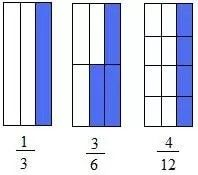2. Jester’s business is currently not doing well. He earned 468 dollars on Friday. He earned 459 dollars on Saturday. He earned 432 dollars on Tuesday. How much did Jester earn on Thursday?
1. 414
2. 424
3. 451
4. 314
3. Study the base-10 blocks below and choose the number it represents. Look carefully before choosing an answer!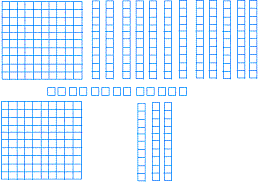1. 164
2. 363
3. 300
4. 452
4. Round 745 to the nearest hundred _____________ and round 2755 to the nearest thousand _____________
5. Look at the options below and choose the number that is the same as five thousand twenty-two.
1. 502
2. 5200
3. 5022
4. 5202
6. Write the decimals 0.4 and 0.25 as fractions and in words.

0.4 :______________   ______________

0.25 : ______________   ______________

7. Which of the answers below is greater than 5668 but less than 5705?
1. 5707
2. 5677
3. 5800
4. 0
8. Look at the equation below and write the value of the symbol # ________________

6 + # = 14

9. How many blocks of 100 and 10 can you create with 3250?
1. 32 and 5
2. 325 and 1
3. 3 and 55
4. 30 and 50
10. 2 nickels, 3 dimes, 6 cents, 1 quarter equals:
1. \$0.71
2. \$0.70
3. \$0.75
4. \$0.69
11. What is the result when you multiply 235 by 13 ________________
1. 3078
2. 4322
3. 566
4. 3055
12. Which of the fractions below is greater than 2/3?
1. 4/5
2. 1/3
3. 2/6
4. 4/6
13. Write 4 multiplication problems that are the same as 12 + 12 + 12
14. What is the shaded fraction of the figure below?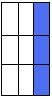1. four-eighths
2. two-ninths
3. nine-three
4. Three-ninths
15. Solve the additions and subtractions below

63827 + 4238 = ?

93335 – 91312 = ?

16. What would you call a  geometric figure that has the shape of a dumpster? ______________________
17. Which of the shapes below could you use to describe the correct shape of an eyeball?
1. Sphere
2. Cube
3. Cone
4. Cylinder
18. Which of the following shapes could you probably use to describe the shape of the refrigerator in your house?
1. Cube
2. Sphere
3. Cylinder
4. Rectangular prism
19. Assuming you have 5 dimes, 3 quarters, 2 pennies, and 1 nickel in your bag. You asked a friend to pick one of the coins. Which coin do you think your friend is most likely to pick? Briefly explain why.
20. Write out the following numbers in words.

5559 ______________

8655 ______________

21. Solve the following long division problems.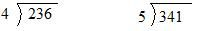22. Which of the two circles below will produce a whole when added together?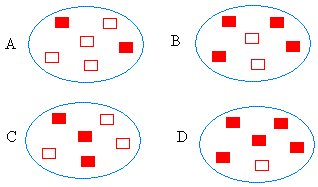1. Choice #1: B and C
2. Choice #2: A and B
3. Choice #3: A and C
4. Choice #4: B and D
23. Draw a circle. Divide the circle into 4 equal parts and shade two-fourths of the circle.
24. Draw two circles. Divide each circle into 4 equal parts. Shade one-fourth of the first circle and three-fourths of the second circle.

a) What is three-fourths plus one-fourth? _______________________

b) What is three-fourths minus one-fourth?_________________________

25. Replace the symbols # and * with numbers to balance the following equation

6 + * = 10 + # + #

26. Which math problem(s) is exactly the same as 40 + (100 + 300)? ______________
1. 40 + 500
2. 140 + 300
3. 40 × 11
4. 40 ×100 + ( 30 )
27. Problem solving skills. Do not use a calculator to solve this problem!

You have 6700 dollars in your bag. You want to buy 7 laptops each costing 800 dollars.

How much will you pay for the 7 laptops?_________

Explain how you got the answer using the multiplication table and math facts.

After paying for the calculators, how much money are you left with in your bag?_________

Using math facts, explain how you got the answer very quickly.

You decide to share the leftover money evenly between you and 10 of your friends. How much money will each person get?____________

28. If 10 books cost 200 dollars, what is the price of 2 books? (Assuming you bought 10 of the same book)
29. What is the area of the shape below?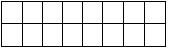1. 10 square units
2. 14 square units
3. 17 square units
4. 16 square units
30. In the space provided below, draw 2 lines that are parallel and another line that is perpendicular to the two parallel lines.
31. In the space below, draw 2 congruent shapes and 2 similar shapes:
32. Complete the sequence below with the next 2 numbers

4, 20, 100, ________, ___________

## What Math Skills Are Required to Pass the 4th Grade Math Test?

The 4 grade math tests will test your abilities in the following areas:

• Geometry: To pass the math benchmark test 4th grade, your child needs to be familiar with geometry topics like lines, angles, identification of line segments, basic geometrical shapes, and polygons.
• Decimals and Fractions: The 4th grade state test math questions will include multiplication and division operations and the questions on how to represent decimals and fractions.
• Measurements: Maths test 4th grade questions will include problems on how to use measurements and problems involving area, length, weight, and area.
• Operations: Students will be tested on addition, subtraction, multiplication, and division.

## Look Online for More Math Help

If your child takes the diagnostic test but doesn’t perform well, there’s no need to panic. All you need to do is get them the help they need to improve their performance. This is where Brighterly comes in.

Brighterly is an online learning website that offers one-on-one math lessons with experienced teachers for kids. This platform uses interactive lessons and fun-based learning methods to help kids learn math faster and improve their perception of learning math. You can apply for Brighterly’s evaluation lesson today to help your child perform better in math tests for 4th grade.

Winter Break Math Camp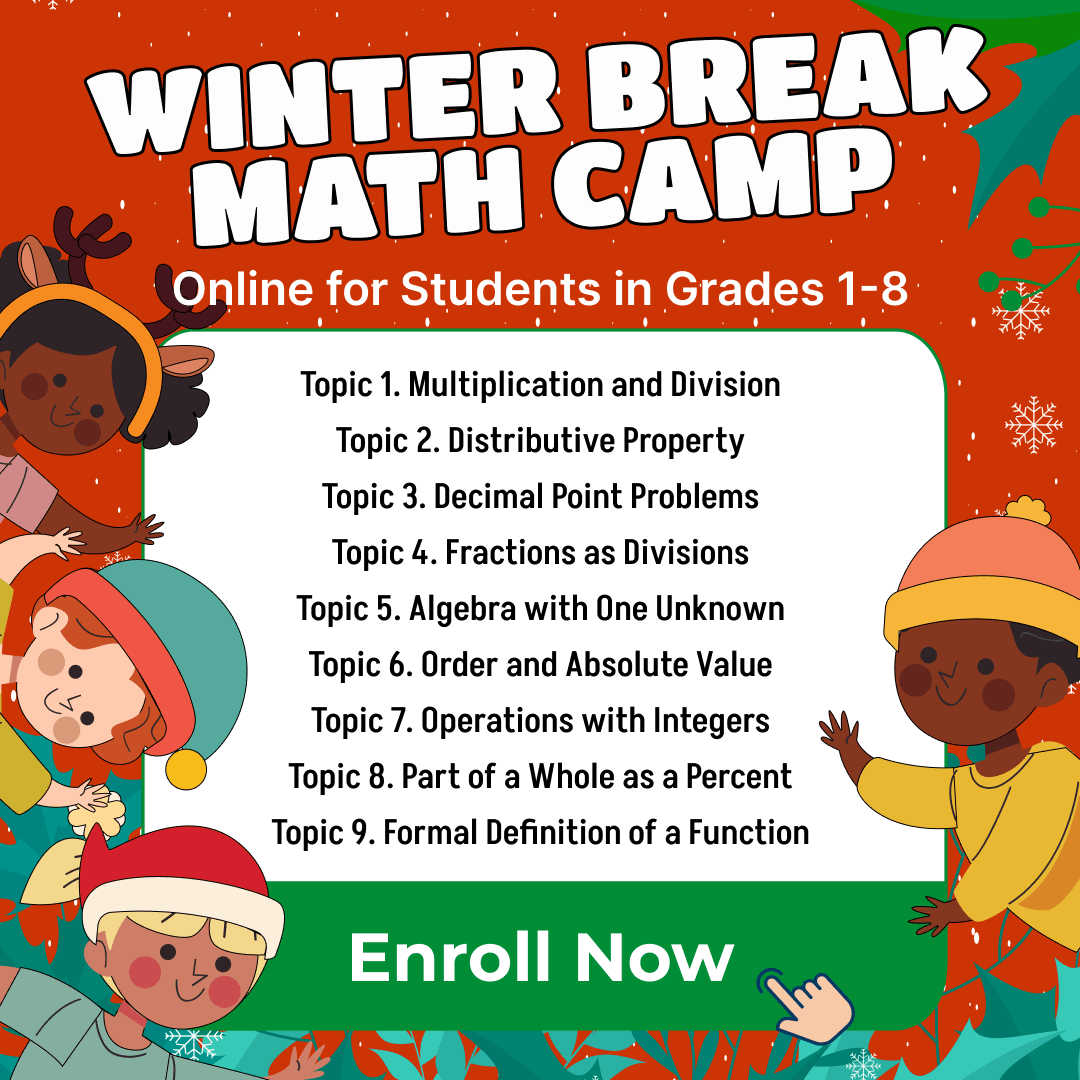• Boost Math Skills this Winter Break at our Camp, Perfect for Students in Grades 1-8!

Winter Break Math Camp
Enhance Math Skills with Our Program, Perfect for 1st-8th Graders, Aligned with School Curriculum!